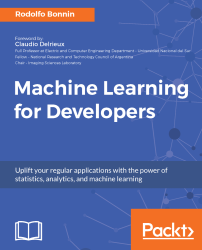•#### Machine Learning for Developers#### Overview of this book

Most of us have heard about the term Machine Learning, but surprisingly the question frequently asked by developers across the globe is, “How do I get started in Machine Learning?”. One reason could be attributed to the vastness of the subject area because people often get overwhelmed by the abstractness of ML and terms such as regression, supervised learning, probability density function, and so on. This book is a systematic guide teaching you how to implement various Machine Learning techniques and their day-to-day application and development. You will start with the very basics of data and mathematical models in easy-to-follow language that you are familiar with; you will feel at home while implementing the examples. The book will introduce you to various libraries and frameworks used in the world of Machine Learning, and then, without wasting any time, you will get to the point and implement Regression, Clustering, classification, Neural networks, and more with fun examples. As you get to grips with the techniques, you’ll learn to implement those concepts to solve real-world scenarios for ML applications such as image analysis, Natural Language processing, and anomaly detections of time series data. By the end of the book, you will have learned various ML techniques to develop more efficient and intelligent applications.
PrefaceFree Chapter
Introduction - Machine Learning and Statistical ScienceThe Learning ProcessClusteringLinear and Logistic RegressionNeural NetworksConvolutional Neural NetworksRecurrent Neural NetworksRecent Models and DevelopmentsSoftware Installation and Configuration# Regression analysis

This chapter will begin with an explanation of the general principles. So, let's ask the fundamental question: what's regression?

Before all considerations, regression is basically a statistical process. As we saw in the introductory section, regression will involve a set of data that has some particular probability distribution. In summary, we have a population of data that we need to characterize.

And what elements are we looking for in particular, in the case of regression? We want to determine the relationship between an independent variable and a dependent variable that optimally adjusts to the provided data. When we find such a function between the described variables, it will be called the regression function.

There are a large number of function types available to help us model our current data, the most common example being the linear, polynomial...